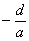3.三次方程

[x31=0] 方程 x31=0

[x3+px+q=0(卡尔丹公式)] 方程

x3+px+q=0

x1=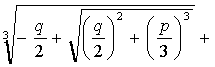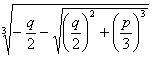x2=ωω2(2)

x3=ω2ωx1+x2+x3=0,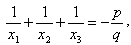x1x2x3=q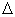=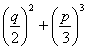>0时，有一个实根和两个复根；=0时，有三个实根，当p=q=0时，有一个三重零根；当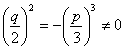时，三个实根中有两个相等；<0时，有三个不等的实根.

x1=2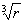cosθ

x2=2cos(θ+120°)

x3=2cos(θ+240°)

r=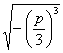, θ=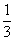arc cos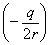[ax3+bx2+cx+d=0] 一般三次方程

ax3+bx2+cx+d=0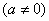x=y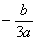y3+py+q=0

x1=y1x2=y2x3=y3x1+x2+x3=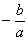,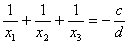, x1x2x3=